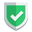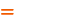我们相信：世界是美好的，你是我也是。平行空间的世界里面，不同版本的生活也在继续...

`neo4j`可以通过`match`语句匹配节点和关系，这里衍生了一个子语句叫做`optional match`，也就是可选的`match`。如何理解这个可选`match`呢？这就是本文中主要阐述的内容。

## 基本数据情况

``match (m)-[r:FOLLOWS]->(n) return m,r,n``

``match (m{name:'James Thompson'}) return m``

## 关注了谁

``match (m{name:'James Thompson'})-[r:FOLLOWS]->(n) return m,r,n``
``match (m{name:'James Thompson'}) match (m)-[r:FOLLOWS]->(n) return m,r,n``

``match (m{name:'James Thompson'}) optional match (m)-[r:FOLLOWS]->(n) return m,r,n``
``optional match (m{name:'James Thompson'})-[r:FOLLOWS]->(n) return m,r,n``

## 被谁关注了

``match (m{name:'James Thompson'})<-[r:FOLLOWS]-(n) return m,r,n``
``match (m{name:'James Thompson'}) match (m)<-[r:FOLLOWS]-(n) return m,r,n``

``match (m{name:'James Thompson'}) optional match (m)<-[r:FOLLOWS]-(n) return m,r,n``

``optional match (m{name:'James Thompson'})<-[r:FOLLOWS]-(n) return m,r,n``

## 综述如果本文对您有帮助，或者节约了您的时间，欢迎打赏瓶饮料，建立下友谊关系。本博客不欢迎：各种镜像采集行为。请尊重原创文章内容，转载请保留作者链接。【福利】 腾讯云最新爆款活动！1核2G云服务器首年50元！【源码】本文代码片段及相关软件，请点此获取更多信息【绝密】秘籍文章入口，仅传授于有缘之人neo4j    cypher